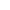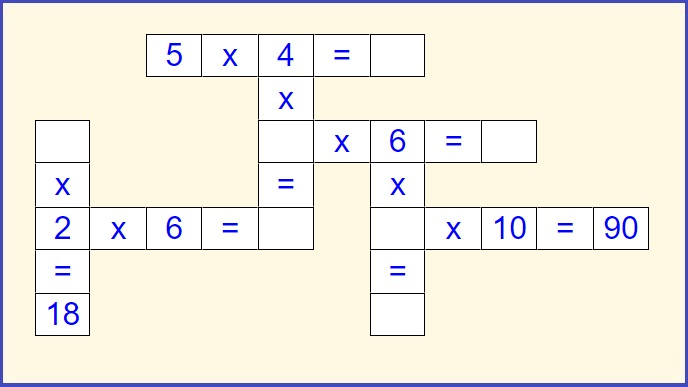﻿ Multiplication crossword puzzles | printable

Page No. 1059

# Multiplication crossword puzzles

Solve multiplication crossword puzzles online. Download free printable multiplication crossword puzzle PDF. Multiplication crosswords are super engaging multiplication games that teach multiplication in a fun way. You can print out multiplication crossword puzzle worksheets PDF. Multiplication math crossword improves logical thinking. For home schooling download multiplication crossword puzzle with answers. crossword puzzle multiplication.

New gameFill in the blanks of each crossword puzzle to make the multiplication equations true.

PRINT WORKSHEET »

## Multiplication crossword puzzles with answersFill in the missing numbers so the operations in multiplication crosswords are correct.

Press "Check it" to find out if the multiplication and division crossword puzzle is filled in correctly. The correct answers will be green, wrong results will be red. Press the button "New game" and the new multiplication math crossword will be generated.

## Free printable multiplication crossword puzzle PDF

These online multiplication crossword puzzles complement well with following multiplication crossword puzzle worksheets. Everytime new different multiplication crosswords are generated, the second page contains answers.

Click the image bellow and download the free printable multiplication crossword puzzle in PDF:

You can find more free printable multiplication worksheets here.

## How to solve multiplication crossword puzzle?

First, look for an operation that you can finish. With the number found you can solve another equation. Continue to do this until all operations are solved and the multiplication crossword puzzle is completed. Each equation should be solved from left to right and from top to bottom in the multiplication crossword puzzle.### Multiplication crossword puzzles

In these multiplication crosswords you are not only looking for results of multiplication equations but also for missing factors.

If you are not sure how to solve multiplication crosswords correctly, press "Check it" button first and you will see answers. You can also watch this video on YouTube.

## Multiplication and division crossword puzzle

These mixed multiplication and division crossword puzzle are super warm-up activity, which can be used during the first few minutes of math class to keep student engaged for the entire lesson.

Because of mixed multiplication and division tasks you can use these multiplication crossword puzzles for teaching single digit multiplication and division at the same time.

### Multiplication crosswords

Learning multiplication facts is always a struggle for so many and something I hear from parents and teachers over and over again. So I thought it would be good to create these math multiplication crosswords.

Looking for ideas for how to teach and build a conceptual understanding of multiplication? Check these printable multiplication games, which include lots of fun ideas and engaging practice games that will help kids make sense of multiplication.